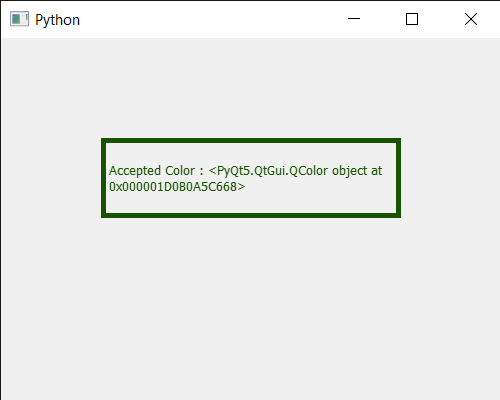GeeksforGeeks App
Open AppBrowser
Continue

# PyQt5 QColorDialog – Accepted Signal

In this article, we will see how we can get the accepted signal of the QColorDialog widget. This signal is emitted when the dialog has been accepted either by the user or by calling accept() or done() with the QDialog::Accepted argument. Note : This signal is not emitted when hiding the dialog with hide() or setVisible(false). This includes deleting the dialog while it is visible. In order to do this we use accepted method with the QColorDialog object

Syntax : dialog.accepted.connect(lambda: print(“Accepted Signal”) Argument : It takes method as argument Action Performed : It will call the passed method when accepted signal emitted

Below is the implementation

## Python3

 `# importing libraries``from` `PyQt5.QtWidgets ``import` `*``from` `PyQt5 ``import` `QtCore, QtGui``from` `PyQt5.QtGui ``import` `*``from` `PyQt5.QtCore ``import` `*``import` `sys`  `class` `Window(QMainWindow):` `    ``def` `__init__(``self``):``        ``super``().__init__()` `        ``# setting title``        ``self``.setWindowTitle("Python ")` `        ``# setting geometry``        ``self``.setGeometry(``100``, ``100``, ``500``, ``400``)` `        ``# calling method``        ``self``.UiComponents()` `        ``# showing all the widgets``        ``self``.show()`  `    ``# method for components``    ``def` `UiComponents(``self``):` `        ``# creating a QColorDialog object``        ``dialog ``=` `QColorDialog(``self``)` `        ``# setting current color to the dialog``        ``dialog.setCurrentColor(Qt.red)`  `        ``dialog.accepted.connect(``lambda``: ``print``("accepted Signal"))` `        ``# making color done``        ``# accepting the color``        ``dialog.done(``1``)` `        ``# executing the dialog``        ``dialog.exec_()`  `        ``# creating label``        ``label ``=` `QLabel("GeeksforGeeks", ``self``)` `        ``# setting geometry to the label``        ``label.setGeometry(``100``, ``100``, ``300``, ``80``)` `        ``# making label multi line``        ``label.setWordWrap(``True``)` `        ``# setting stylesheet of the label``        ``label.setStyleSheet("QLabel"``                            ``"{"``                            ``"border : ``5px` `solid black;"``                            ``"}")` `        ``# getting the selected color``        ``color ``=` `dialog.selectedColor()` `        ``# setting graphic effect to the label``        ``graphic ``=` `QGraphicsColorizeEffect(``self``)` `        ``# setting color to the graphic``        ``graphic.setColor(color)` `        ``# setting graphic to the label``        ``label.setGraphicsEffect(graphic)` `        ``# setting text to the label``        ``label.setText("Accepted Color : " ``+` `str``(color))`   `# create pyqt5 app``App ``=` `QApplication(sys.argv)` `# create the instance of our Window``window ``=` `Window()` `# start the app``sys.exit(App.``exec``())`

Output :

```Accepted Signal
Accepted Signal```My Personal Notes arrow_drop_up